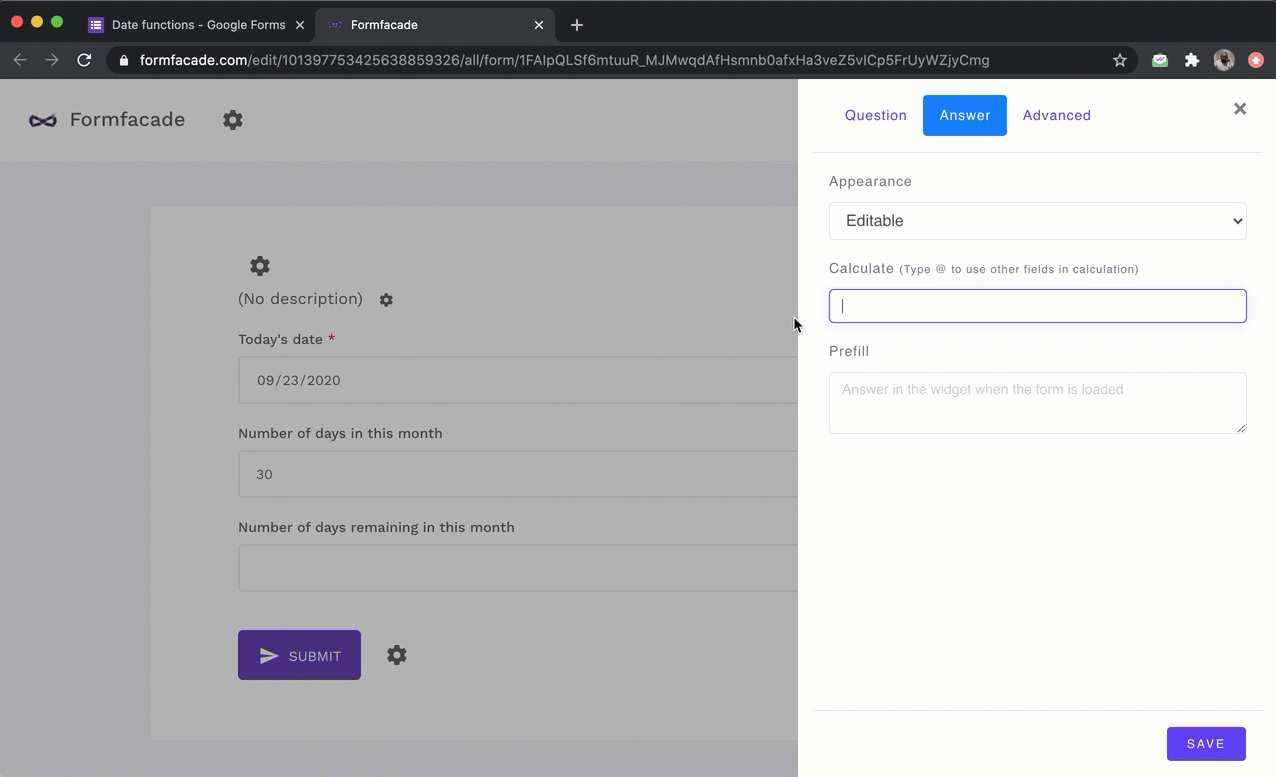# How to calculate the number of days left in a month?

### You can calculate the number of days in the month using DATE function and then subtract the current day of the month to calculate the days remaining in that month.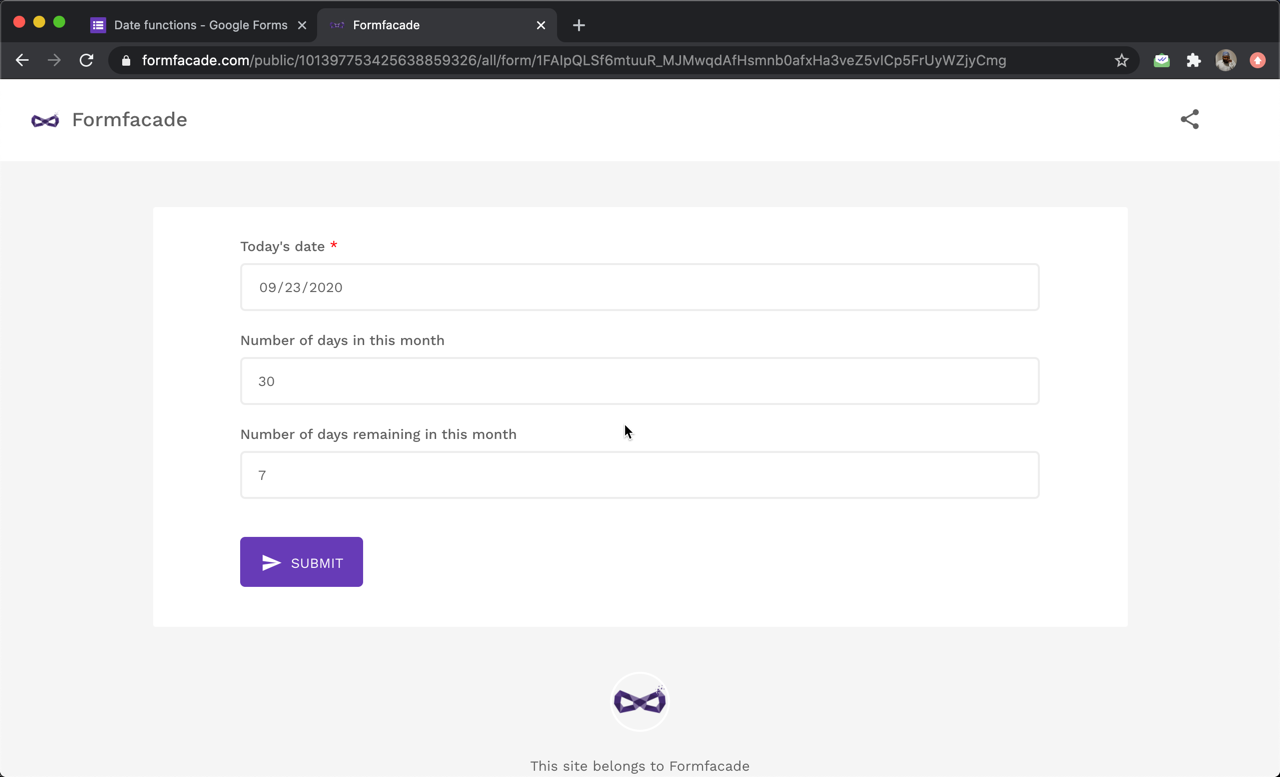#### How to calculate the number of days in this month?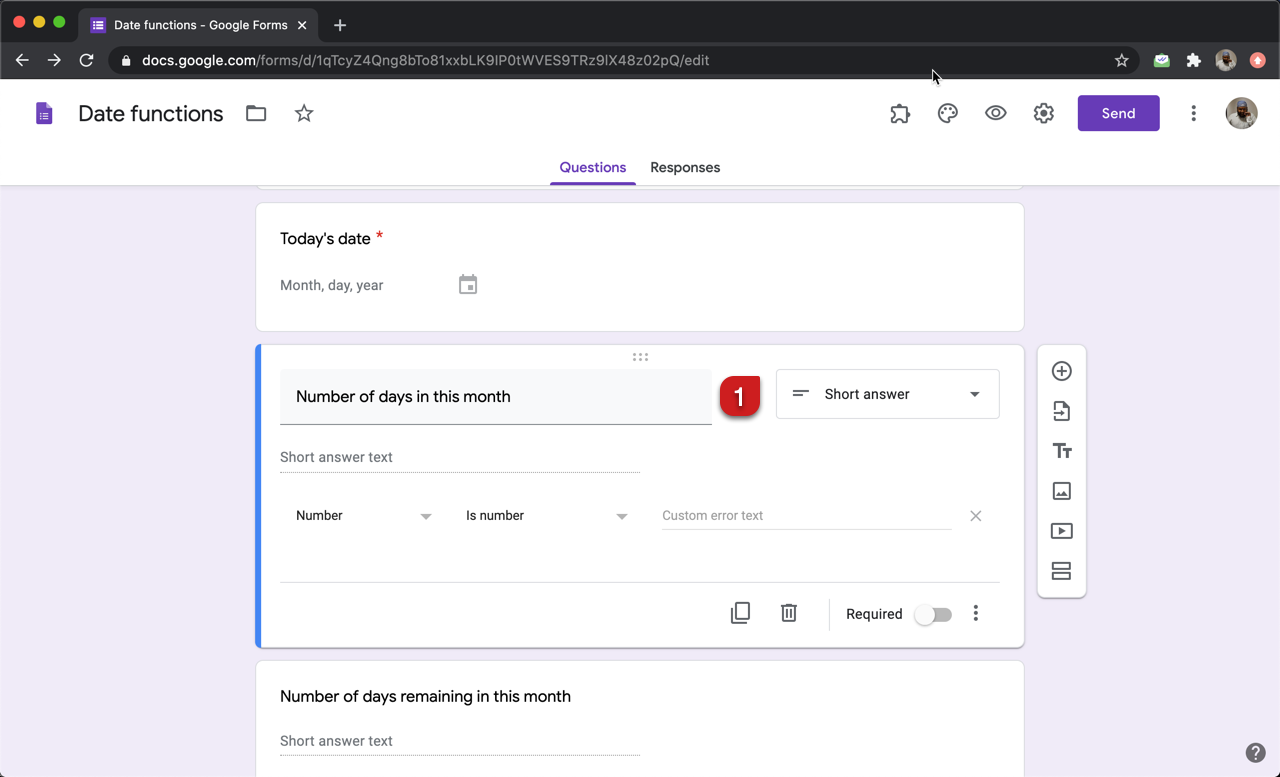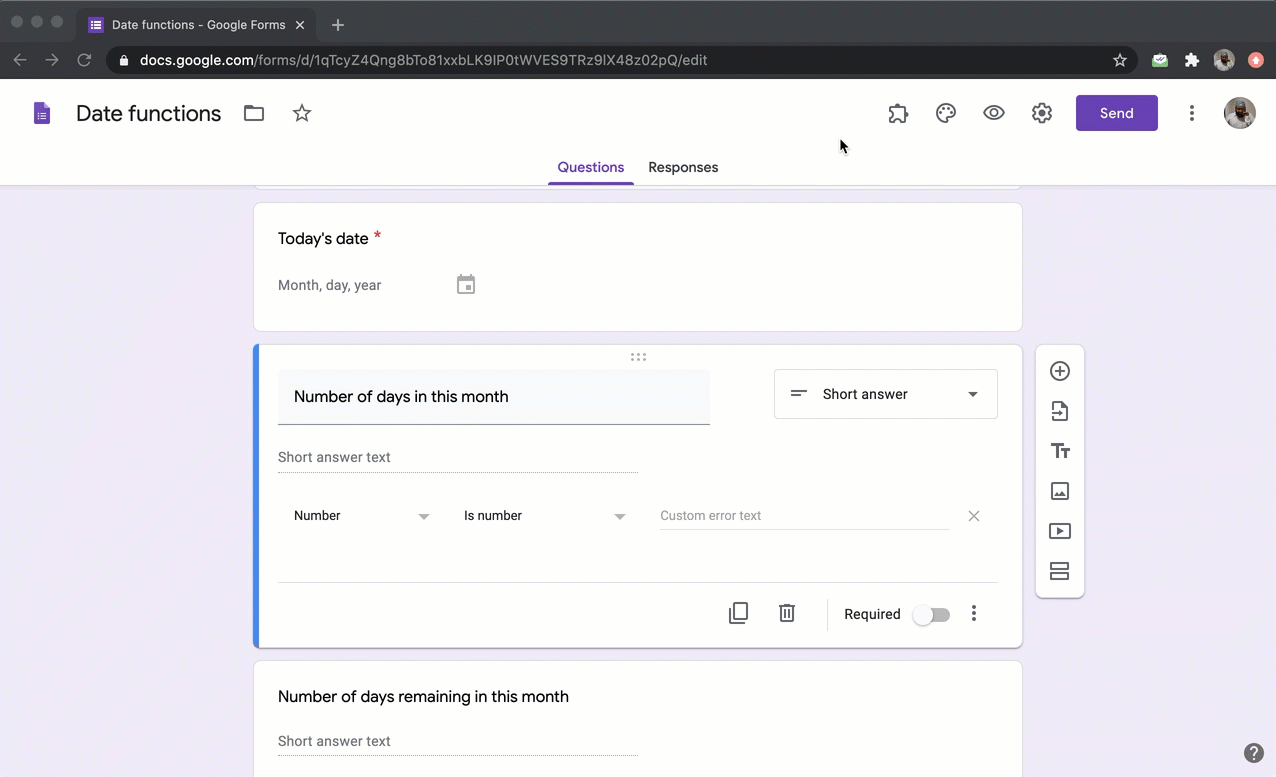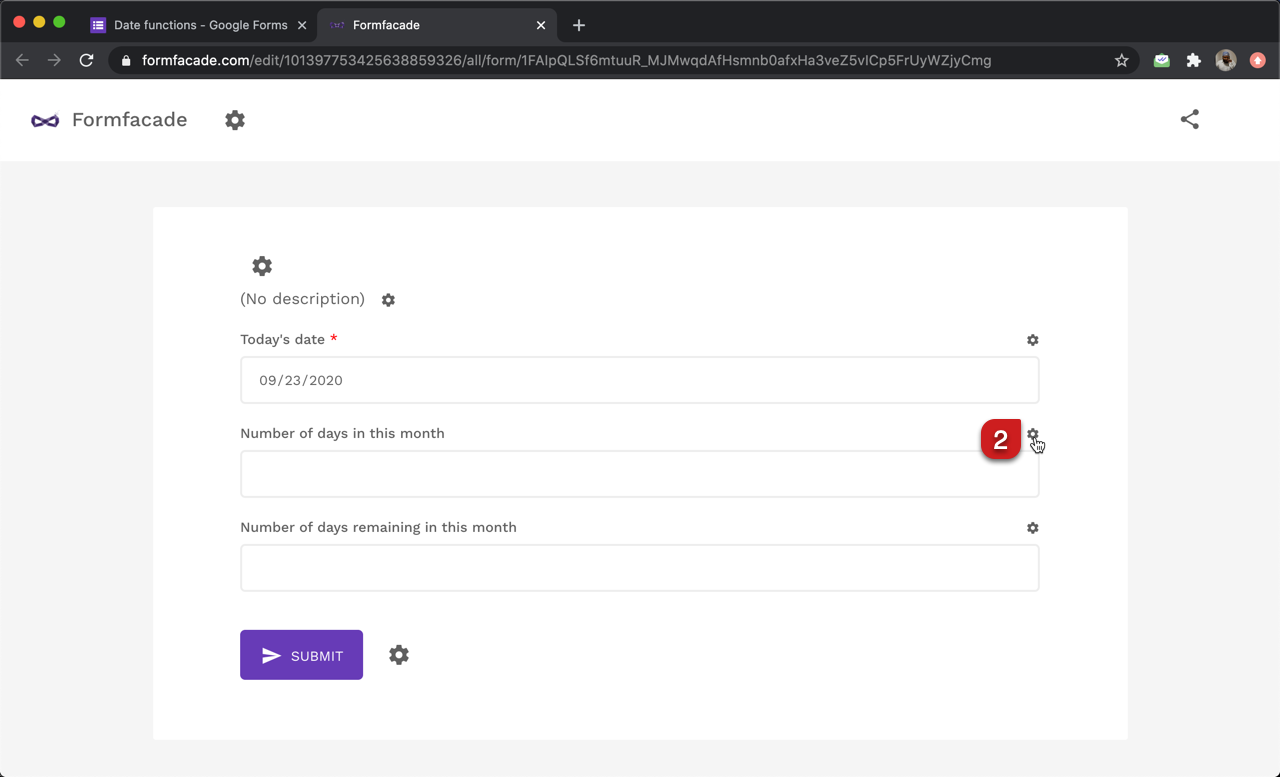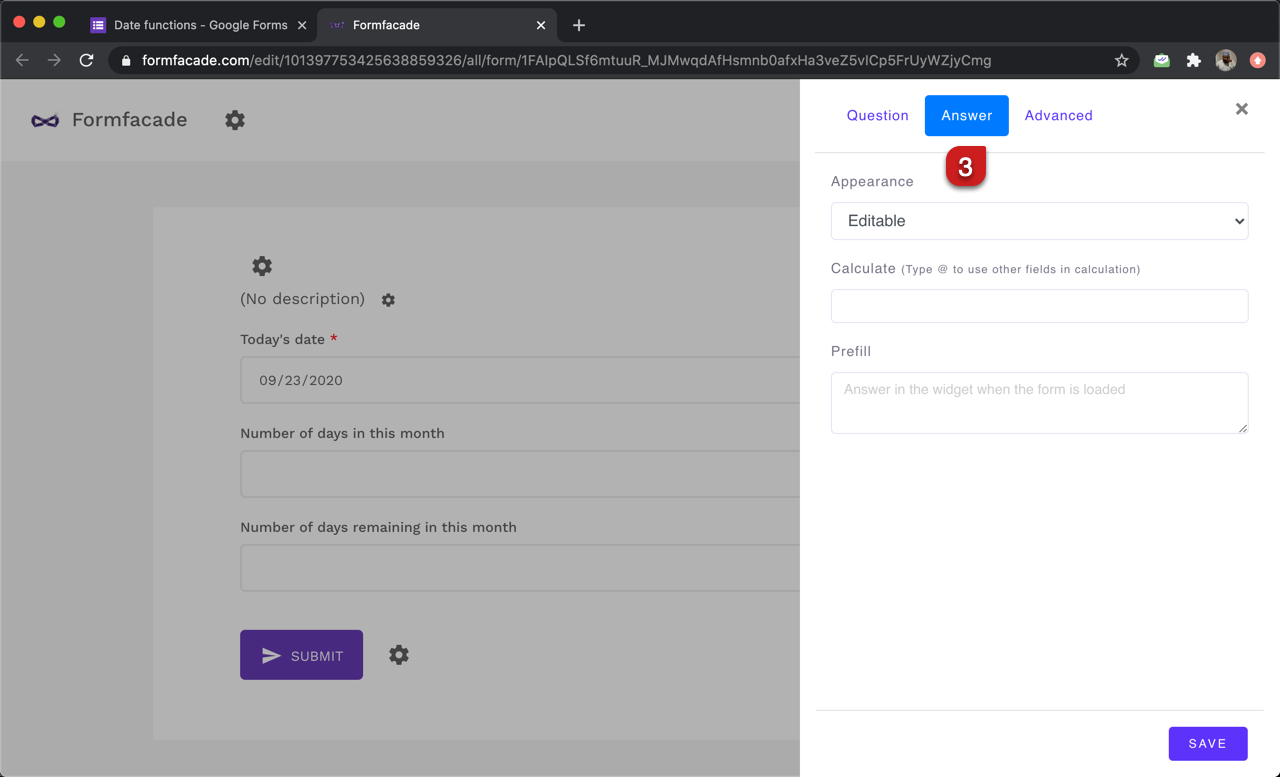#### Use DATE function to calculate the days in this month

The first step is to calculate the last date of this month. We can pass the year, month and day to the date function.

TODAY().year() will return the current year
TODAY().month() will return the current month
DATE(TODAY().year(), TODAY().month()+1, 1) will return the first day of next month.

We can then use the SUBTRACT function to find the last day of this month.
DATE(TODAY().year(), TODAY().month()+1, 1).subtract(1,"days")

There is another way to calculate the last day of this month without using the SUBTRACT function.
DATE(TODAY().year(), TODAY().month()+1, 0) will return the last day of this month.

We can use the getDate() or date() function to find the day of the month for a specified date.
DATE(TODAY().year(), TODAY().month()+1, 0).date() will return the last day of the month.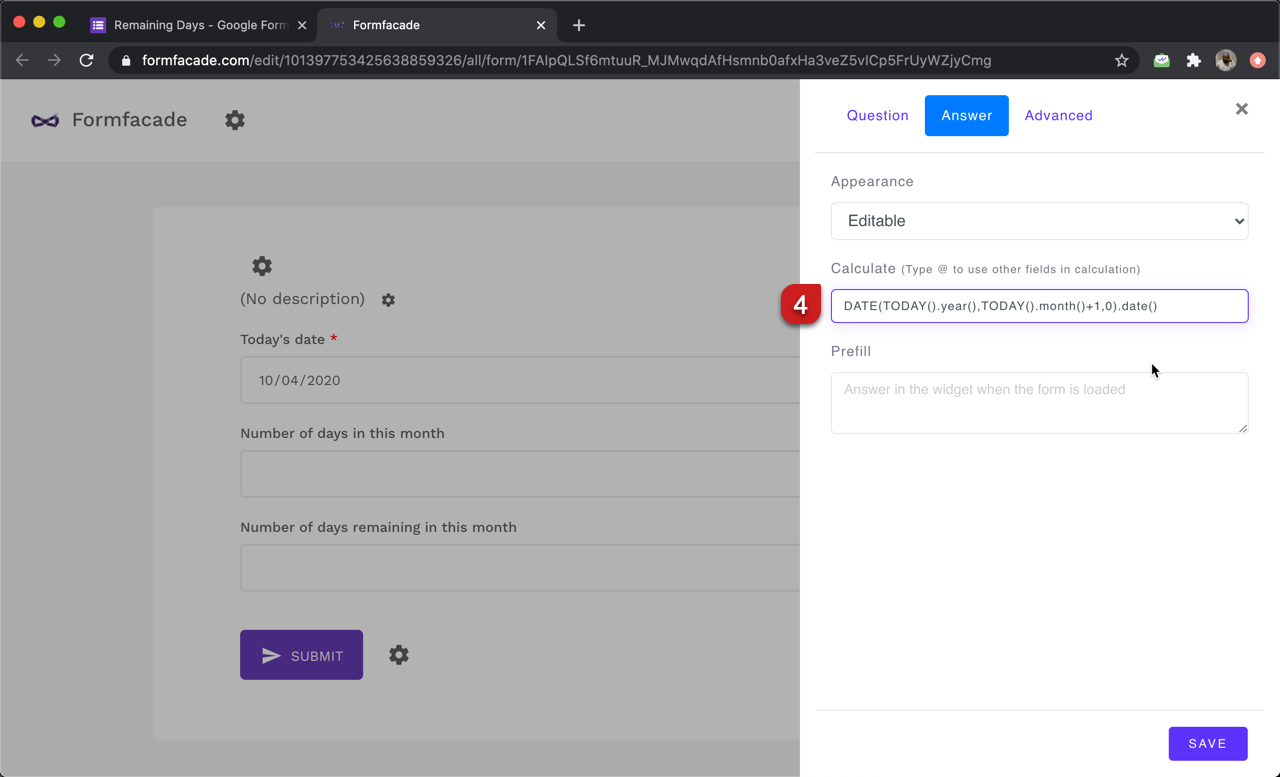#### How to calculate the number of days remaining in this month?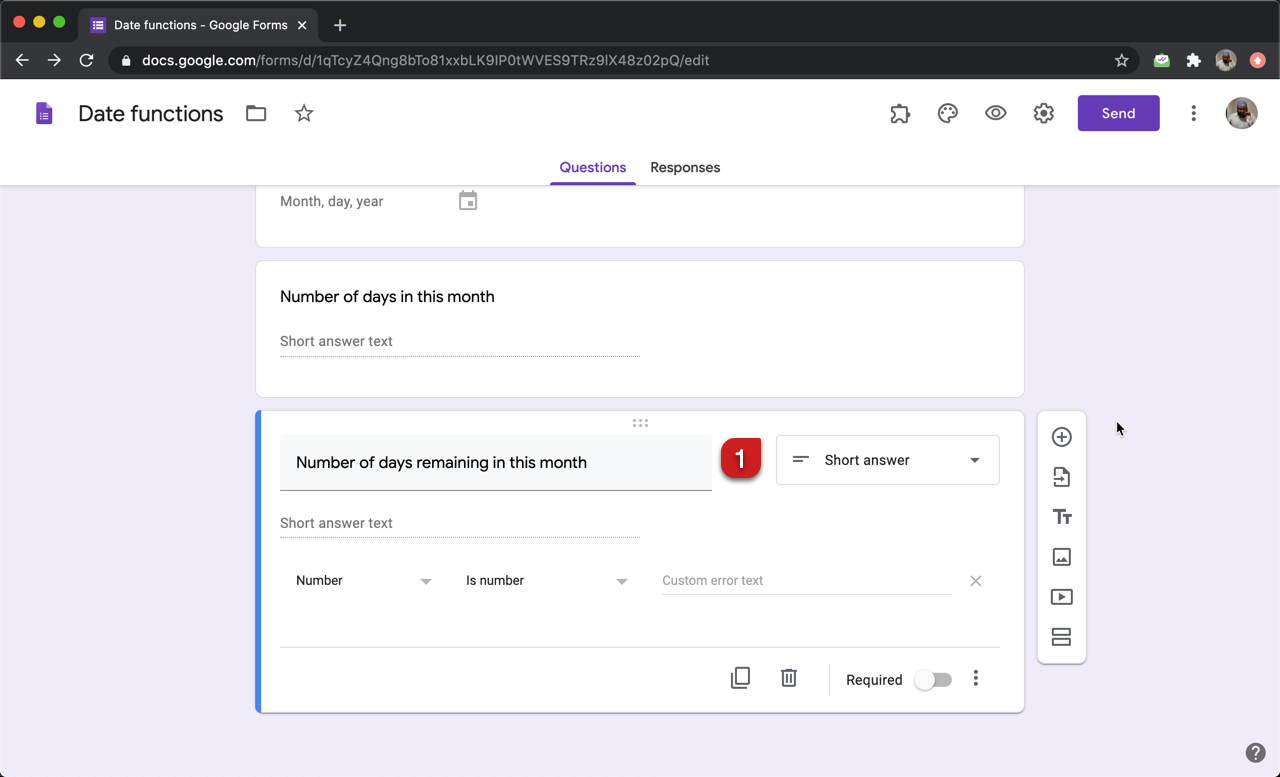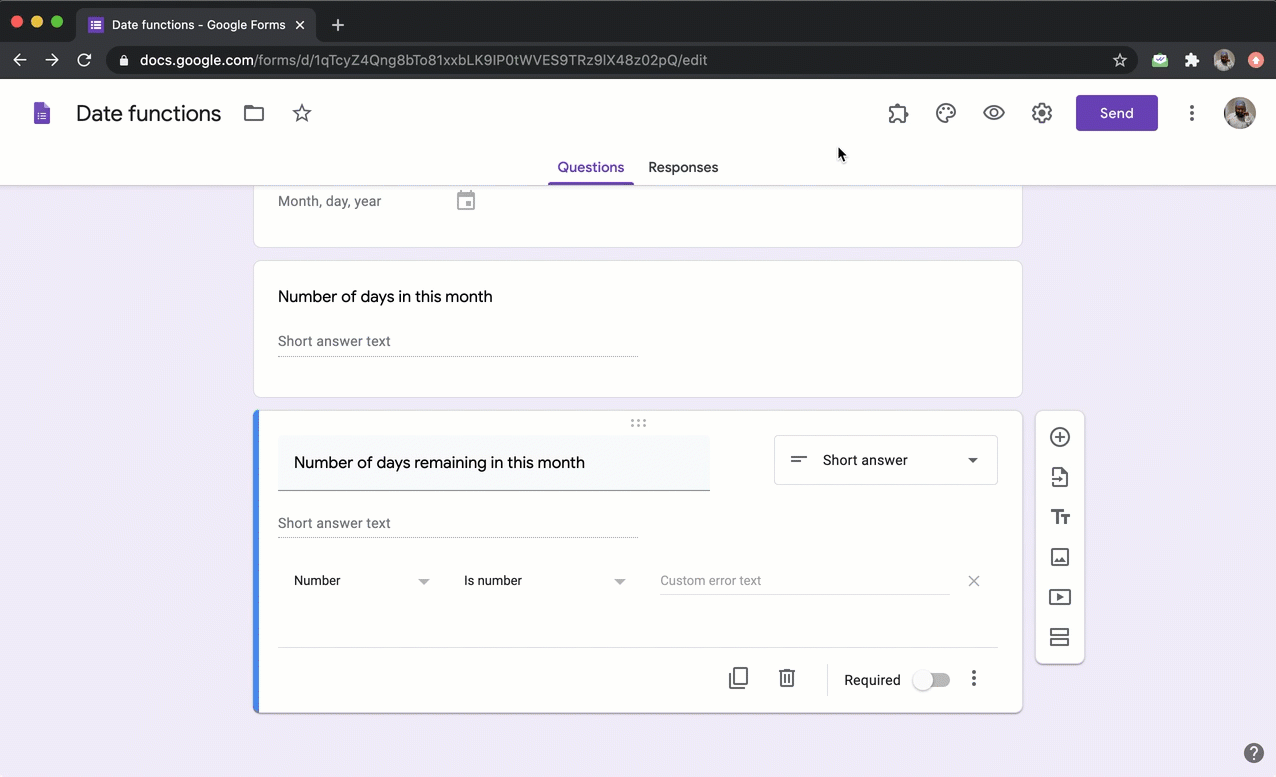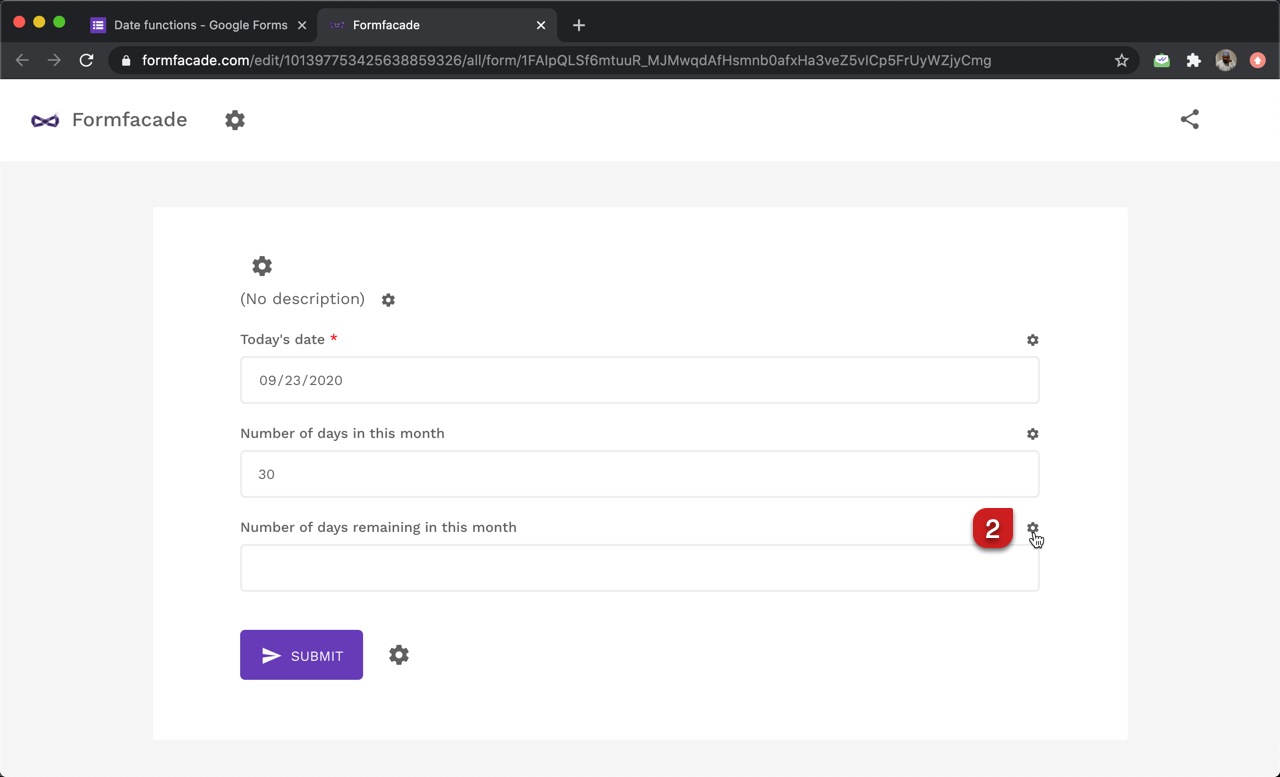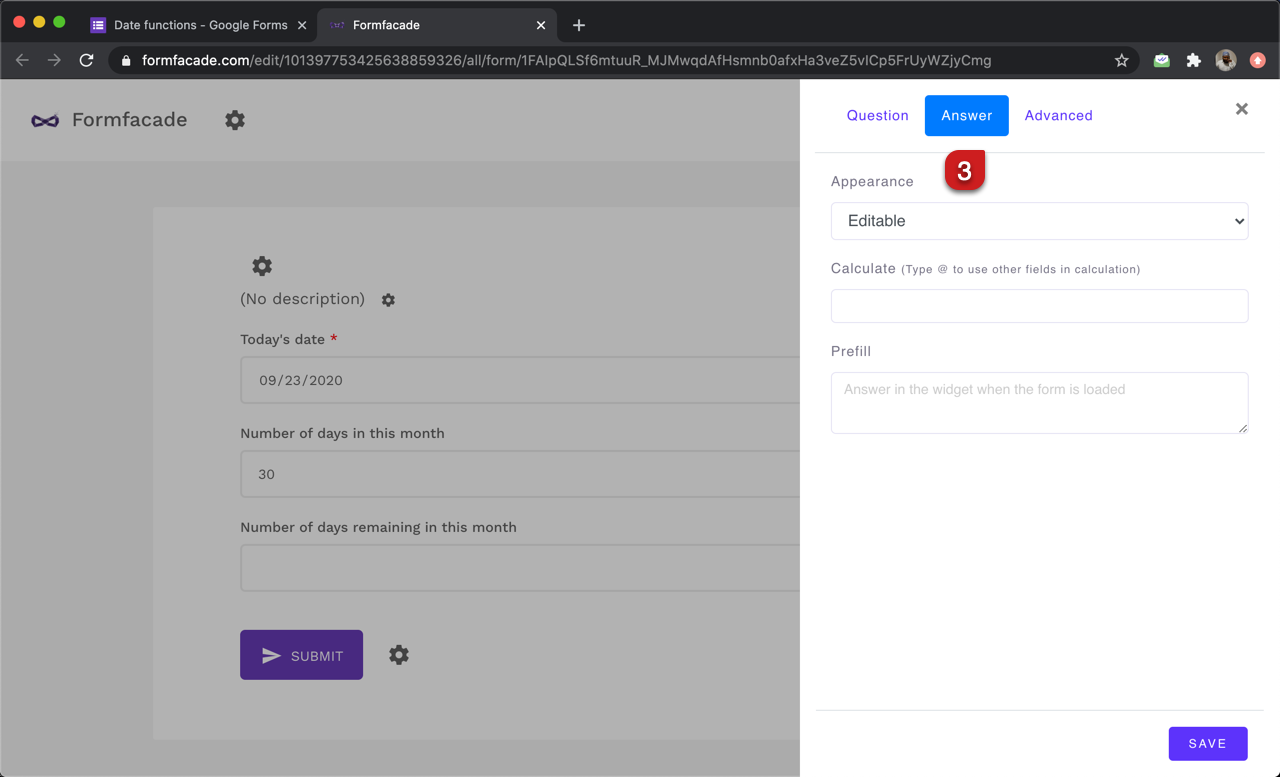#### Use DATE function to find the day of this month

We can use the getDate() or date() function to the find day of the month for a specified date. TODAY().date() will return the current day. To calculate the remaining days in the month, we can use the below formula.

DATE(TODAY().year(), TODAY().month()+1, 0).date() - TODAY().date

Since we have already calculated the number of days in this month as an answer for a question in the form, we can refer that instead of writing the formula again.

days_in_this_month - TODAY().date()

Note: To enter days_in_this_month, type @ in the calculate option and select the number of days in this month question from the list.